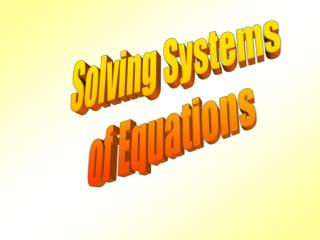DownloadDownload PresentationSolving Systems of Equations

# Solving Systems of Equations

Télécharger la présentation## Solving Systems of Equations

- - - - - - - - - - - - - - - - - - - - - - - - - - - E N D - - - - - - - - - - - - - - - - - - - - - - - - - - -
##### Presentation Transcript

1. Solving Systems of Equations

2. We have seen previously that a system of two equations in two unknowns has only 3 possible solution sets. A unique solution; exactly one ordered pair (r, s) that satisfies both equations. The graphs of the corresponding lines intersect once. Infinitely many solutions; the graphs of the corresponding lines are identical. No solutions; no ordered pair (r, s) satisfies both equations. The graphs of the corresponding lines do not intersect. The two lines are parallel.

3. Intersecting Lines y = x + 5 y = -x -1 2 = -(-3) – 1 2 = 3 – 1 2 = 2  2 = -3 + 5 2 = 2  This screen shows two lines which have exactly one point in common. The common point when substituted into the equation of each line makes that equation true. The common point is (-3,2). Try it and see.

4. Coinciding Graphs y = -2x + 2 y = -6/3x +2

5. Parallel Lines Line 1 crosses the y-axis at 3 and has a slope of 3. Therefore, the equation of line 1 is y = 3x + 3. Line 2 crosses the y-axis at 6 and has a slope of 3. Therefore, the equation of line 2 is y = 3x + 6. Parallel lines have the same slope, in this case 3. However, parallel lines have different y-intercepts. In our example, one y-intercept is at 3 and the other y-intercept is at 6. Parallel lines never intersect. Therefore parallel lines have no points incommon.

6. Example Use any method to solve the system below x – y = 2 3y + 2x = 9

7. You Try It Graph the system of equations. Determine whether the system has one solution, no solution, or infinitely many solutions. If the system has one solution, determine the solution.

8. Some in-class problems. You choose the method. • x + 3y = 10 –x + 2y = 5 2. 2x - 3y = 10 5x + 2y = 7 3. 5x + 10y = 10 x + 2y = 2 4. 0.5x - y = 5 x – 0.5y = 4

9. Classwork/Homework